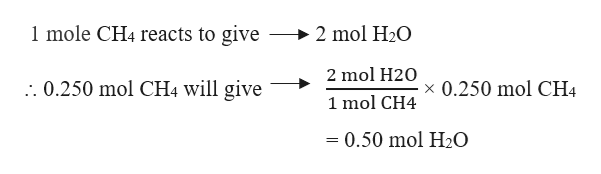# theoretical yield of chemical formulas

Question
1 views

theoretical yield of chemical formulas

check_circle

Step 1

The theoretical yield is the amount predicted by a stoichiometric calculation based on the number of moles of all reactants present in a balanced chemical reaction.

In case of more than one reactant, the theoretical yield is calculated from the limiting reactant. The limiting reactant is the reactant in the chemical reaction which limits the amount of product that can be formed.

Step 2

Let us take a problem where it is asked to calculate the theoretical yield.

Example : A mixture of 0.250 mol of methane was burned in 1.25 mol of oxygen in a sealed steel vessel to give water and carbon dioxide. Find the theoretical yield of water in grams.

Solution:

Firstly, we need to write a balanced chemical reaction. The balanced reaction will be:

CH4  + 2 O2 ------>  CO2 + 2 H2O

Step 3

Now, to find the theoretical yield of water, first we have to find out the limiting reactant. This can be done by comparing the actual molar ratio of the reactants given in the problem with the stoichiometric ratio from the balanced reaction.

Stoichiometric ratio (CH4 : O2) = 1 : 2

Actual molar ratio (CH4 :...help_outlineImage Transcriptionclose1 mole CH4 reacts to give 2 mol H2O 2 mol H20 : 0.250 mol CH4 will give 0.250 mol CH4 1 mol CH4 = 0.50 mol H20 fullscreen

### Want to see the full answer?

See Solution

#### Want to see this answer and more?

Solutions are written by subject experts who are available 24/7. Questions are typically answered within 1 hour.*

See Solution
*Response times may vary by subject and question.
Tagged in

### Chemistry